# Understanding Resources and Costs

 <  Day Day Up  >

The most important resources for a project are the people who do the work, but you also have to provide those people with the facilities in which to do the work and the equipment and materials they need to do their jobs. The cost of a project is usually based on the sum cost of all the resources needed to complete each task.

#### Defining Resources

The term resource refers to the people and assets that must be assigned to work on a task until it is completed. Resources include workers, supervisors, managers, the plant and equipment, facilities, supplies , and materials. You might also choose to assign as resources people who don't actually work on the task or whose work you do not need to measure (such as outside contractors or vendors ), but whose name you want associated with the task. The list of resources available for work on a project is known as the resource pool .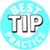Resources that are necessary to complete activities within a project typically fall into three categories. People are the most common resource category tracked and are usually listed one of three ways. The first way is by name; the second way is by type, which can be listed in the pool as a skill set; and the third way is by outsourced vendor, providing an indication of accountability or responsibility for the task. The next most common category of resources tracked is equipment. Sometimes use of a truck, crane , or computer might be necessary to complete work within a project. Often, use of a facility, such as a training room or testing lab, falls into this category of resource. Finally, materials and supplies comprise the third category of resources that is often closely monitored .

##### Defining the Work and Material Resource Types

Microsoft Project distinguishes two resource types : work resources and material resources. Work resources contribute their time and effort (that is, their work) to a task during the time they're scheduled to work on a task, but when the task is completed, they have not been consumed by the task and they have future time that they can devote to other tasks . People, facilities, and equipment are examples of work resources. Material resources , on the other hand, are consumed or used up when they are assigned to a task and are no longer available to be assigned to other tasks. Lumber, concrete, and camera film are examples of material resources. Chapter 9, "Understanding Resource Scheduling," explains the way Project handles resource availability and costs for both of these resource types.

##### Defining Single and Grouped Resources

Some resources that you add to a resource list represent individual people or assets. You can use a person's name, for example, as a resource name, and you can name a single piece of equipment, a facility, or a raw material as a resource. If you outsource a task to a vendor who is totally responsible for the task, you could name the vendor as a resource name (even though there might be many individuals on the team who actually work on the task).

You can also define a group resource (what Microsoft Project sometimes calls consolidated resources ) to represent multiple identical or interchangeable resources. These could be individual people with identical skills or multiple pieces of identical equipment. For example, you might define a group of five electricians as the Electricians resource and a set of four forklifts as the Forklifts resource. With a consolidated resource, you are not concerned (in your project plan) with which individual resource is assigned to a given task. You let the manager in charge choose the individuals to do the work.

CAUTION

All the individuals in a consolidated resource must share a common cost rate and must be scheduled by a single resource calendar that is defined for the group. You cannot assign unique cost rates to individual members of a consolidated resource, and you cannot recognize individual vacation days or other nonworking times for the individuals.

##### Defining Generic and Placeholder Resources

When multiple individual resources share a certain skill set or capacity, and you haven't decided which specific individual to assign to a specific task, it's useful to create a generic resource to represent that specific pool of talent. For instance, if you have a staff of 20 engineers and are planning a new project, you could create a resource called Engineers as a generic resource and assign the Engineers resource to the individual tasks. Later you can substitute the names of specific engineers in the task assignments. This method has the advantage of letting you calculate how many engineers you will need on the project without committing individuals to specific tasks. You will see in the section "Grouping Resources," later in this chapter, how you can aggregate the assignments for a generic resource such as Engineers with the assignments for individual engineers to calculate the total usage of engineers in the project.

NOTE

Using generic resources to forecast resource needs is especially important when many project managers share a common resource pool. When contemplating a new project, you should add a draft of the project to see exactly what impact it will have on resource needs.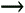If you use the Professional edition of Project 2003, you can automatically optimize the substitution of resources with similar skill-sets. Identifying generic resources is key to that process. See "Using the Resource Substitution Wizard," p. 1110 .

#### Defining Costs

Project stores and calculates a number of measures of cost. It is important that you understand what the different terms mean so that you can provide the required information that will enable accurate cost calculations.

##### Resource Costs, Fixed Costs, and Total Costs

Most of the costs of a project are due to the cost of using resources to complete the project, and these costs are called resource costs . The cost of each work resource assigned to a task is generally based on the hours of work or effort associated with the assignment and the hourly cost of using the resource. If a task's duration changes, then its hours of assigned work and the cost of the resource assignment will change as well. Therefore, Project adjusts costs as you revise a project schedule. For material resources, the cost is based on the cost of each unit of the material and the number of units assigned to the task.

If there are costs that are not specifically related to any one named resource, you can attach the amounts of those costs directly to the task. These costs are called fixed costs .

The sum of the resource costs and fixed costs is called total cost .

##### Resource Cost Calculations

When you assign a work resource to a task, Project calculates the cost of the task by multiplying the cost rate per unit time for the resource by the amount of time the resource spends on the task. Thus, if a work resource costs \$10 per hour and it spends two hours on a task assignment, Project calculates \$20 as the cost of that assignment. If you assign multiple resource names to a task, the sum of all the individual resource assignment costs is totaled as the resource cost of the task.

Material resource costs, on the other hand, are based solely on the number of units consumed or used up by the task, and are only indirectly, if at all, based on the duration of the task. If the material resource goes directly into the output of the task, like lumber goes into framing a house, then the duration of the task has no effect on the number of units of material required for the task. The material cost is simply the number of units of the resource (the board feet of lumber) multiplied by the per-unit cost of the resource (the cost per board foot of lumber).

If the material resource is consumed by a work resource, like diesel fuel is consumed by a bulldozer, the number of units consumed can be affected by the duration of the task. The longer the task, the more hours of work required from the work resource (the bulldozers) and therefore the more material resource (the fuel) that is needed. Using the bulldozer as an example, the material cost is calculated by multiplying the number of units consumed per unit of time (that is, the gallons of fuel per hour) by the cost per unit (that is, the cost per gallon).

##### Fixed Costs

If there are costs that are incurred in completing a task but you don't want to define a resource to associate the cost with, you can treat the cost as what Project calls a fixed cost . The legal fees associated with a contract or construction permit fees could be treated as fixed costs. But if you want to keep track of the legal fees or permit fees, you should create an appropriately named resource and assign it to the task. A fixed cost is entered in the task table as a lump-sum amount.

TIP

You should always document the reasons for the fixed cost and how the amount is determined in the task Notes field. This is especially important in the event that you hand the project over to someone else to manage.To learn the steps you take to actually assign fixed costs to tasks, see "Assigning Fixed Costs and Fixed Contract Fees," p. 396 .

##### Total Cost

Together, the resource costs and the fixed costs add up to the total cost. The field name is just Cost, but it is titled Total Cost in many views. Figure 8.2 shows how assignment costs, fixed cost, and total cost are related. The Gantt Chart view with the Cost table is displayed in the top pane and the Task Form with the details for Resource Cost is shown in the bottom pane. Note that the column titled Total Cost in the top pane is the total cost for the task, whereas the column titled Cost in the bottom pane is the cost of the individual resources assigned to the task.

##### Figure 8.2. The Cost table displays Fixed Cost and Total Cost, with the difference between them being the cost of resources.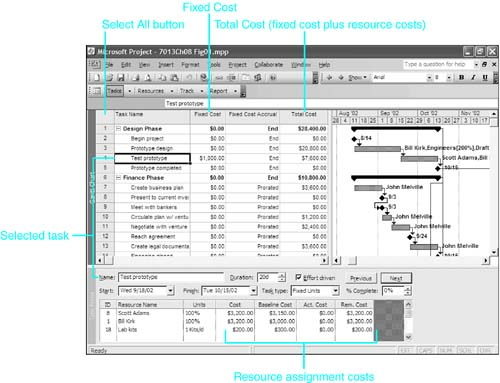To display the Cost table, right-click the Select All button and click Cost on the shortcut menu.

In Figure 8.2, the Test Prototype task is selected in the top pane. Its fixed cost and total cost appear in the top pane, and the details for the assigned resources are shown in the bottom pane.

The cost of the work by Bill Kirk and Scott Adams plus the cost of the lab kits brings the resource cost to \$6,600.00 for the task. There is also a \$1,000.00 fixed cost for the task, which brings the task's total cost to \$7,600.00.

##### Scheduled Costs, Baseline Costs, Actual Costs, and Cost Variances

The total cost described in the preceding section (that is, the sum of resource and fixed costs that is displayed in the Cost field) is calculated as part of the current schedule and is sometimes also called the scheduled cost or the current cost . At the end of the planning stage, just before you start the actual work on the project, the project schedule contains the final projections or estimates of what the project should cost (which your budget should reflect). You must remember to use the Save Baseline command to make a copy of these final cost estimates in the Baseline Cost field (also called the budgeted costs ). You will use the baseline cost later for benchmark comparisons with actual costs.

The scheduled costs will probably change because of revisions in the schedule after work gets under way. For example, if you find that a task is going to take longer to finish than originally estimated and you change the duration of the task in the schedule, Project calculates new scheduled costs because of the additional work that the resources must then complete. But the baseline costs that you have set aside remain unchanged, as a record of the planned cost.

As work is completed on the project, you should track the progress on a regular basis, marking tasks as completed or partially complete, and recording the actual duration and actual work. If the task takes longer than originally scheduled, Project calculates the added costs of the resources. The cost of work that is completed on a task is accumulated in the Actual Cost field. (See the next section, "Accrued Costs," for more information about calculating actual costs.) Project also automatically copies actual costs to the Cost field. The Baseline Cost field retains the original planned costs throughout this process. With actual cost updates added to the Cost field, the scheduled costs now provide a more accurate estimate of the cost of the completed project.

The difference between the Cost field (scheduled cost) and the Baseline Cost field (planned or budgeted cost) is called the cost variance and is stored in the Cost Variance field. The Cost Variance is calculated as Cost minus Baseline Cost. Thus when the variance is a positive number, the schedule is running over budget.

These additional cost fields are displayed in Figure 8.3, in which the vertical divider bar has been moved to the right to display more of the Cost table. In the lower pane, the scheduled cost for the selected task is the column titled Cost and the actual cost is the column titled Act. Cost.

##### Figure 8.3. The Cost table displays the baseline cost, actual cost, and cost variance (the difference between the scheduled and baseline costs).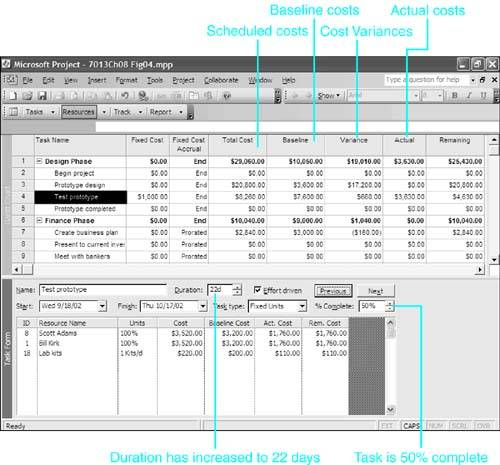To illustrate the baseline and actual costs, some actual work has been recorded in Figure 8.3. Although you don't see a column in the table for the percentage complete, the Prototype Design task has been marked as 100% complete, and its actual cost is now the same as its scheduled and baseline costs, with zero variance. The selected task is Test Prototype and you can see its details in the bottom pane. Before the initial work on Test Prototype was started, its estimated duration was increased from 20 days to 22 days ”a change that has increased the scheduled cost of the resources that are assigned to the task. Also, the work on the Test Prototype task has now been marked as 50% complete.

The increase in the duration of the Test Prototype task in Figure 8.3 has increased the work, and therefore the Cost entries for the assigned resources in the bottom pane. The total of the resource costs has risen from \$6,600 (the sum of the entries in Figure 8.2) to \$7,260 (the sum in Figure 8.3). Project calculated the Actual Cost entries by taking 50% of each of the Cost entries. The sum of the Actual Cost amounts for the resources is \$3,630. Notice that this is the same value that is in the Actual column in the top pane. In other words, the Actual amount includes only resource costs from the bottom pane. Project did not calculate any part of the Fixed Cost as having been incurred (that is, accrued ) at the halfway point in finishing the task. The following section explains why.

##### Accrued Costs

When you mark a task as partially complete, Project must calculate what part of the scheduled cost will be shown as Actual Cost. Until the task is complete, these costs are accrued costs and the way Project calculates them is governed by the accrual method selected for the resources and for the task's fixed cost. By default, if you mark a task as partially complete, Project prorates the scheduled resource costs. In Figure 8.3, for example, the selected task is marked 50% complete and Project has applied 50% of the scheduled resource costs to actual cost. In Figure 8.3, the Fixed Cost Accrual setting for this task is End; Project will therefore apply all \$1,000 of the fixed cost to actual cost only when the task is marked 100% complete.

You specify the accrual method for determining when resource cost will be recognized as actual cost when you define the resource. (See the Accrue At column in Figure 8.1.) You can use one of the following three methods for accruing costs:

• Prorated ” The default accrual method for both material and work resources is the Prorated method. With this method, Project recognizes a prorated portion of the scheduled cost as actual cost based on the percentage complete of the duration for the task.

• Start ” This method recognizes the resource cost at the moment work on the task is scheduled to start. For example, if the resource must be paid in full up front before it starts an assignment, you could recognize the full cost as being expended at the start of the task.

• End ” This method withholds recognition of the cost until the task is completed. For example, if the resource will be paid only if the finished work is satisfactory, you might withhold recognition of the cost until the task is finished.

Note that the choice of the accrual method is significant only when a task has started but is not yet complete. All three accrual methods yield the same result after a task is completed. Thus, only interim cost reports are affected by the accrual method.

NOTE

If you are using timephased cost reporting, the choice of accrual method is important from a reporting perspective. For example, if you report on a daily basis for the task cost using the start method, all task cost is reported on the first day of the task. The total cost for the task is, however, the same with any method when the task is complete.

 <  Day Day Up  >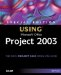Special Edition Using Microsoft Office Project 2003
ISBN: 0789730723
EAN: 2147483647
Year: 2004
Pages: 283
Authors: Tim Pyron

Similar book on Amazon